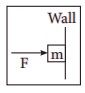# TN 11TH PHYSICS - Online Test

Q1. The center of mass of a system of particles does not depend upon,
Explaination / Solution:
No Explaination.

Q2. If the masses of the Earth and Sun suddenly double, the gravitational force between them will
Explaination / Solution:
No Explaination.

Q3. If a wire is stretched to double of its original length, then the strain in the wire is
Explaination / Solution:
No Explaination.

Q4. The graph between volume and temperature in Charles’ law is
Explaination / Solution:
No Explaination.

Q5. A sample of ideal gas is at equilibrium. Which of the following quantity is zero?
Explaination / Solution:
No Explaination.

Q6. A particle executing SHM crosses points A and B with the same velocity. Having taken 3 s in passing from A to B, it returns to B after another 3 s. The time period is
Explaination / Solution:
No Explaination.

Q7. A transverse wave moves from a medium A to a medium B. In medium A, the velocity of the transverse wave is 500 ms-1 and the wavelength is 5 m. The frequency and the wavelength of the wave in medium B when its velocity is 600 ms-1, respectively are
Explaination / Solution:
No Explaination.

Q8. If the error in the measurement of radius is 2%, then the error in the determination of volume of the sphere will be
Explaination / Solution:
No Explaination.

Q9. Identify the unit vector in the following.
Explaination / Solution:
No Explaination.

Q10. An object of mass m held against a vertical wall by applying horizontal force F as shown in the figure. The minimum value of the force F isExplaination / Solution:
No Explaination.